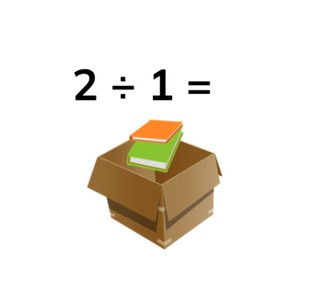Solving the division table of 1

# Solving the division table of 1

Students learn to solve the division table of 1.

No account needed.8,000 schools use Gynzy92,000 teachers use Gynzy1,600,000 students use Gynzy

## General

The students learn to solve the division table of 1.

## Standards

CCSS.Math.Content.3.OA.B.5/6

## Learning objective

Students will be able to solve the division table of 1.

## Introduction

You choose a student volunteer to put the balls on the board in the correct order on the line.

## Instruction

First you discuss the importance of calculating division problems. Next you explain that with a division problem you have a certain number of something in each group and for the division table of 1 you divide each number by 1. For this you show an example of 3 monkeys that must be evenly divided into 1 tree. After this you explain how you solve a division problem by saying how dividing by 1 works with images of books that must be divided into a box. Next you say that with the division table of 1 the answer is actually always the same as the number that you begin with. After this you ask a volunteer student to divide the swans into the pond and then you have the students state the problem that matches the picture. Then you have the students solve abstract problems.

Check whether the students can solve the division table of 1 by asking them the following questions:
- What does the problem 4 ÷ 1 mean? Can you tell an example of this?
- How do you actually always know the answer when solving the 1 division table?

## Quiz

The students test their understanding of solving the division table of 1 through ten exercises. For some of the exercises the students have possible answers to choose from, and for the others they must fill in the answer on their own.

## Closing

You have the students solve one more division problem and after that you do a division problem shoot-out. The students work in a group. One student names a problem from the division table of 1. The others must say the answer as quickly as they can. The person who gave the answer the fastest can say the next division table problem.

## Teaching tips

You can have the students solve division problems with concrete materials by having them make groups of 1.

## Instruction materials

Concrete materials

### The online teaching platform for interactive whiteboards and displays in schools

• Save time building lessons

• Manage the classroom more efficiently

• Increase student engagement